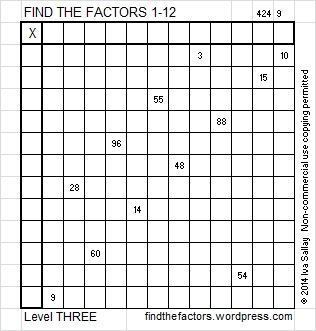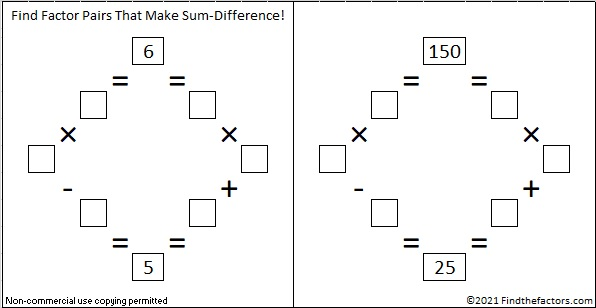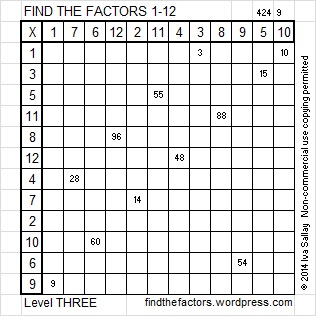# 150 and Level 3

### Today’s Puzzle:### Factors of 150:

Excel file of puzzles and previous week’s factor solutions: 12 Factors 2014-06-16

 150 is a composite number. Factor pairs:150 = 1 x 150, 2 x 75, or 3 x 50, 5 x 30, 6 x 25, or 10 x 15 Factors of 150: 1, 2, 3, 5, 6, 10, 15, 25, 30, 50, 75, 150 Prime factorization: 150 = 2 x 3 x 5 x 5, which can also be written 150 = 2 x 3 x (5^2)### Sum-Difference Puzzle:

6 has two factor pairs. One of those factor pairs adds up to 5, and the other one subtracts to 5. Can you place those factors in the proper boxes to complete the first puzzle?

150 has six factor pairs. One of those factor pairs adds up to 25, and another one subtracts to 25. If you can identify those factor pairs, then you can complete the second puzzle!The second puzzle is really just the first puzzle in disguise.  Why would I say that?

### A Logical Way to Solve Today’s Puzzle:

A Logical Approach to FIND THE FACTORS: Find the column or row with two clues and find their common factor. Write the corresponding factors in the factor column (1st column) and factor row (top row).  Because this is a level three puzzle, you have now written a factor at the top of the factor column. Continue to work from the top of the factor column to the bottom, finding factors and filling in the factor column and the factor row one cell at a time as you go.